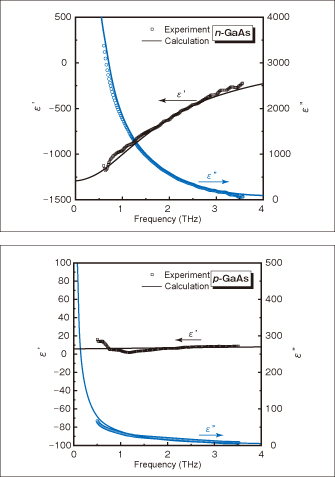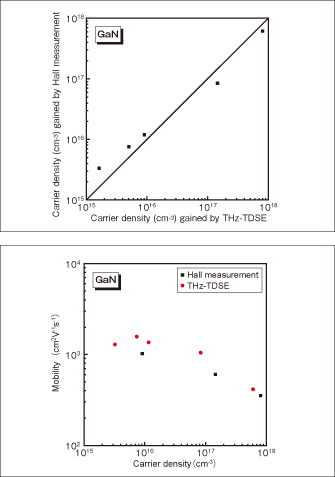# Paper Review 1

## Noncontact Measurement of PhysicalProperties of Semiconductors by Terahertz Time-Domain Spectroscopic Ellipsometry

Figure 3 shows measured results of the permittivity of n- and p-GaAs homoepitaxial thin films formed on a semi insulating GaAs substrate. The doping densities and the film thicknesses are 4 x 1018 cm-3, 1.0µm and 1 x 1018cm-3, 0.5µm, respectively. The solid linecurve in Fig. 3 was worked out by Drude model and shows that the value gained from the experiment matches the calculated value. The carrier density and mobility which were gained from this calculation matched the value gained by Hall measurement within the tolerance of ±10%. With a p type sample, where the entrance length was 5.8µm at 1THz, which was 10 times as large as the sample thickness, satisfactory measurement sensitivity was gained. The THz-TDSE method allows THz light to enter a sample at a shallow incidence angle, thereby being able to gain large polarization variations. Therefore, with a thin film sample, high measurement sensitivities can be gained.Fig. 3 Measured values of complex permittivity of n-type (above) and p-type (below) GaAs homoepitaxial thin films

Figure 4 gives the summary of the measurement of carrier density and mobility of GaN free-standing substrate with different doping levelValues gained with the THz-TDSE almost match the values of the Hall measurement. In this way the method can present electrical-physical properties without contacting a material and imposing large controlling conditions on a sample for analysis.Fig. 4 Measured results of carrier density of GaN free-standing substrate (above) and mobility (below)